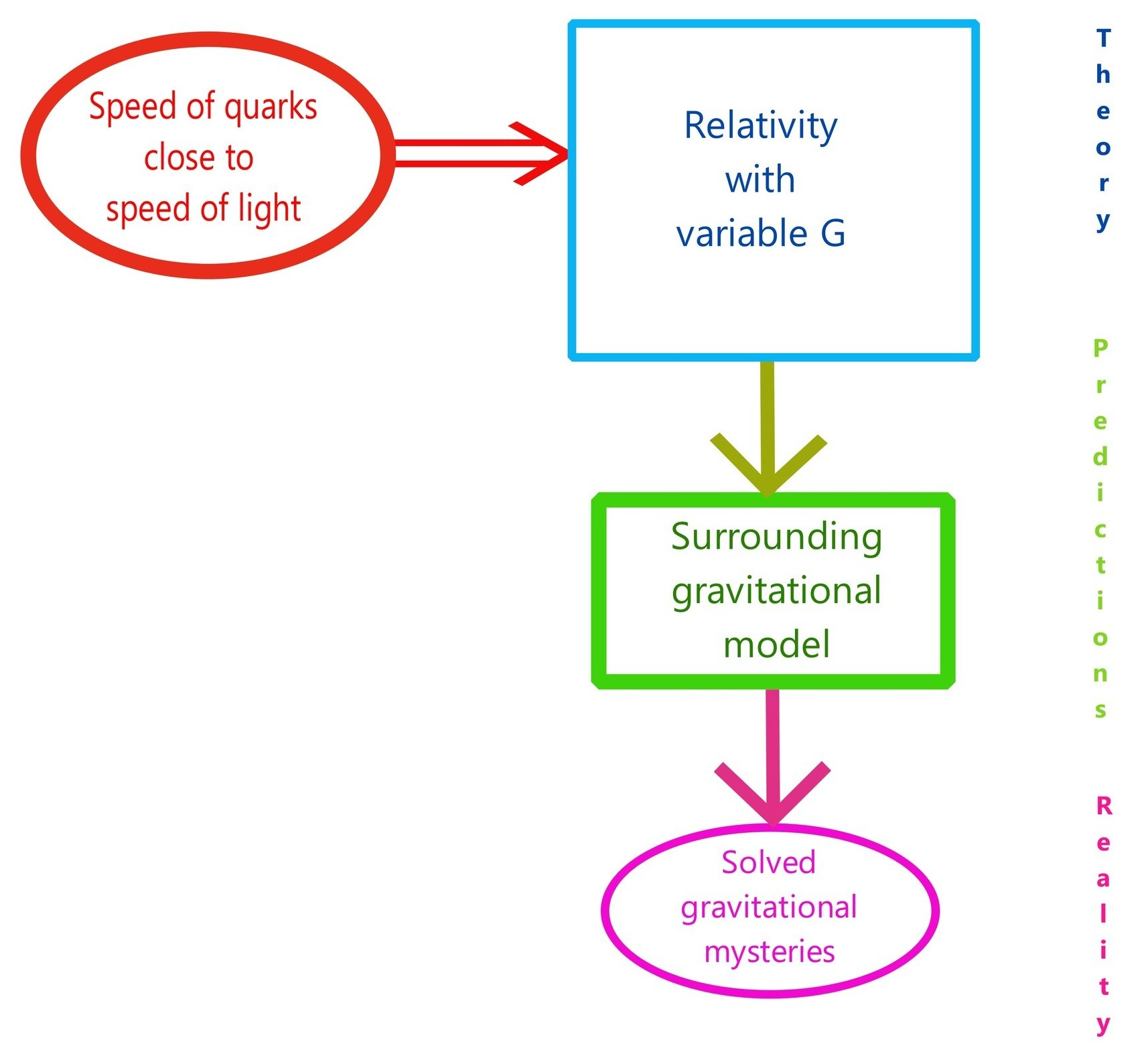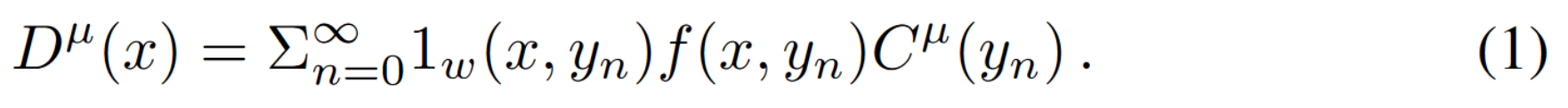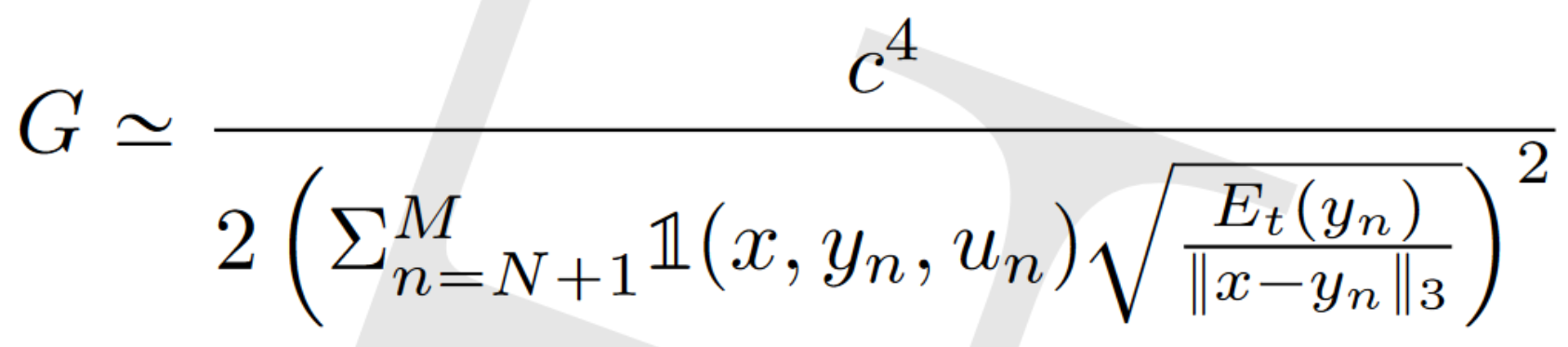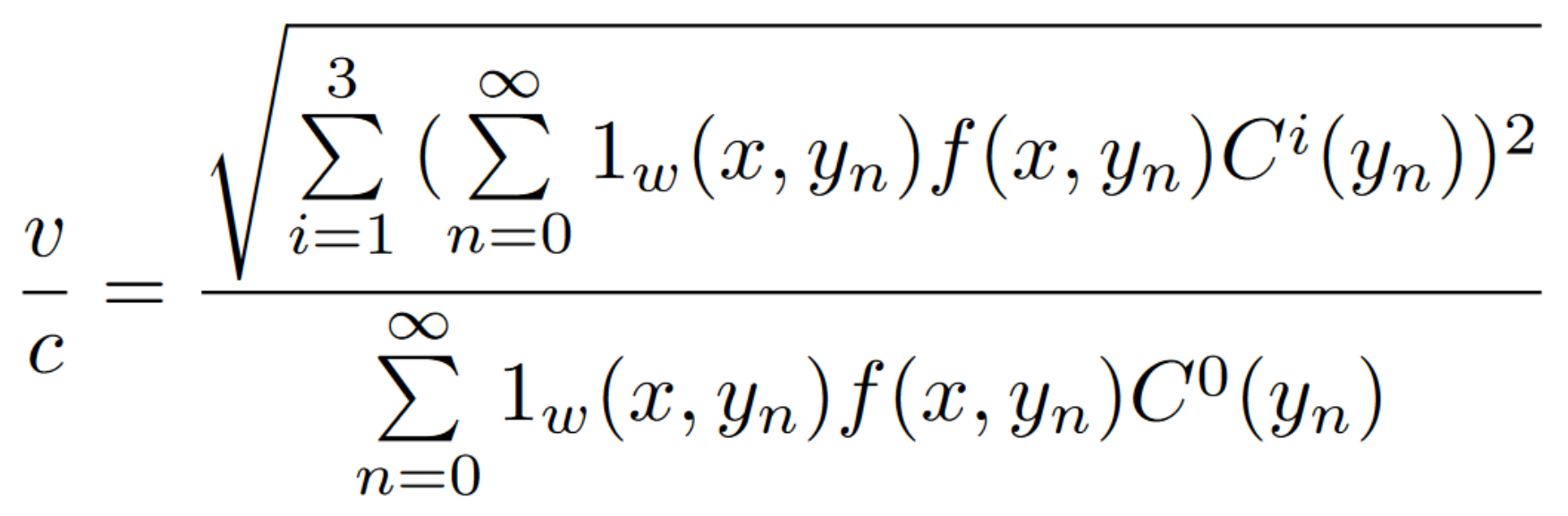04 Nov
04Nov

Relativity must be updated since the discovery of the quasi-relativistic speed of the quarks. From it, the surrounding gravitational model derives.

It means that the calculations of the gravitational predictions of relativity are wrong. And when this slight error is corrected then it remains no gravitational mysteries anymore.

More precisely, this study starts from realizing that in General Relativity the consequences of the speed of the quarks has not been taken into account correctly. It has been established in the 60s that this speed is quasi-relativistic. Hence the quarks generate microscopic gravitational waves, and therefore the gravitational interaction appears now to be retarded. That's the first, immediate, consequence. But this has nearly no gravitational effects, except may be when quasi-relativistic speeds of macroscopic objects are involved, this might appear in cosmology.

Another, more subtle consequence, is the arising of what I called a « surrounding » effect. It appears when taking into account the energy of all the gravitational waves of the quarks. This must be calculated in any space-time event where the gravitational force is calculated. Each gravitational wave energy intervenes in this calculation. It behaves like applying to each space-time event a retarded green function, like in electrodynamics . From this calculation the surrounding effect arises. Roughly speaking this effect is that the energy surrounding this event must divide the Newton's law gravitational potential, after multiplication by an adequate constant. This surrounding effect then gives rise to the surrounding gravitational model. And this one happens to show astonishing agreement to the experimental data of the gravitational mysteries of today.How does this surrounding effect arise from the quark's quasi-relativistic speed ? Well, the involved mechanism can be understood by a thought experiment. Let's imagine a sole wave propagating. Let's call this wave the "A" wave. And the universe is supposed full of such waves, resulting in a macroscopic metric being flat euclidean. If matter density of the universe is weak, each time A will encounter another wave (generated by some other quark in the universe), it will not modify much the space-time deformation which is generated by A. But if matter density of the universe is strong, then there will be more of those encounters, and also the other waves encountering A will get a stronger energy. Those other waves will add also their strong contributions to the final space-time deformation. Therefore the relative contribution of A to this final deformation will be weaker. And finally the final space-time deformation generated by A will be weaker. This retrieves the surrounding effect.

In a second step the study addresses the Millenium Yang-Mills problem of strong force confinement. For that the two following assumptions must be done.

- "Standard model particles are constituted of extremely small amounts of energy allways moving at the speed of light". Those "amounts of energy" do not interact directly with standard model particles. This is only saying that those standard model particles are not solid blocks of matter: inside of them there is energy in motion and no energy at rest. That's all.

- "The four forces are unified by gravity".

From those assumptions, General Relativity now plays a role in particle physics, and the surrounding effect arises in particle physics. This effect appears each time there is a triple interaction between particles, which is the case with the tree quarks of an baryon. This gives a simple explanation to the mystery of the confinement of the strong force.

Below is the published article and the slides of the associated talk done in several conferences in 2022 and 2023.

Link to the article. (Published under its old name "Relativity in motion").

Deprecated versions of this study are still available on the web:  Gravitational Model of the Three Elements Theory (GMTET), Gravitational Model of the Three elements Theory: Formalizing, Surrounding Matter Theory: first mathematical developments , Discrete relativity. Please do not take them into account. Also the name itself has been modified. From "Relativity in motion" it has been renamed "Updated relativity", in 2023. But the old "Relativity in motion" name might still appear on the web. The published version of Updated relativity is too short (15 pages). I had to withdraw important part of this study in order to put it into a 15 pages description. For example, the equation of G is not given. There are still part of the deprecated versions (listed above) which are still correct but which are not in the published article. The next version of Updated relativity will be a long version incorporating everything.

The remaining question is : "why such a big mistake has been done for so long, in the understanding of the gravitational predictions of relativity ?"

Many answers might be given, such as, may be, the fact that particle physics and gravitation has been different fields of research without real collaboration between them.

In that respect, the official science point of view is enlightening (here the particle physics point of view given by wikipedia):

« Gravitation is too weak to be relevant to individual particle interactions except at extremes of energy (Planck energy) and distance scales (Planck distance). However, since no successful quantum theory of gravity exists, gravitation is not described by the Standard Model. »

So this is the reasoning in particle physics, which is of course very much correct. But in contrast, in gravitation, no reasoning is given for explaining why the motion of particles does not modify macroscopic gravitation. It means that the only existing reasoning is the immediate one. It is that an energy of motion is strictly equivalent to an energy at rest: E=mc². But this equation must be used with caution when approximating things!Let's detail how the correct reasoning is paved with traps.

Einstein equation relates matter densities (in its relativistic shape which is a stress energy tensor) to space time curvature. Here is the first trap. Because of this use of matter densities, we assume that only macroscopic scale can be dealt with in relativity. And that is of course, wrong. Einstein can and must be applied at any scale. But here, for those who happens to go over the first trap, comes the second trap. Because now that we understood that the quarks are generating gravitational waves, we try to imagine what is the macroscopic effect of that. And we immediatly have in mind the E=mc² equation. And then we stop thinking. But let's suppose that we still go over this second trap. Then we are left with 2 processes. The first process is the following. We apply E=mc² to a set of quarks, let's say say a hadron, at rest in some frame. Then we apply Einstein equation on this hadron. The second process is the following. We calculate the gravitational waves of those quarks, and we get a space time deformation which is the mean value of the space-time deformations coming from those waves. Same result. And we think that the 2 processes yields the same result. The problem here is that this is true (!). So we stop thinking. This was the third trap and we have fallen in it with ease. We have forgotten that the feature of the quasi-relativistic speed of the quarks must be taken into account for each hadron in the universe. Taking this into account, we still see the same result! Because we iterate the same reasoning as above, for each hadron. This was the fourth trap. Because the mean value can't be calculated that way. In any space-time event, all the gravitational waves coming from all the hadrons of the universe must be taken into account, all at once. We realize that it is forbidden to make the approximation too early, for each hadron. Now the calculation is done for all the waves at once. But now if we only use Einstein equation at this step it will be extremely cumbersome. We have to calculate the space time metric resulting from those numerous gravitational waves, which are interfering with each other. This is a technical difficulty. I overcomed it in my article by using an approximating equation obtained by considering the energies of the gravitational waves. This approximating equation uses a retarded green function, like in electrodynamics. But the fifth trap here, in front of this difficulty, is to imagine that the final result will be the same as before when we have done the approximation too early for each hadron. But this is wrong, it is not the same result. Because now the surrounding effect arises. The involved mechanism giving rise to this effect has been illustrated above, please refer to the paragraph about the "sole A wave", above. So there are 5 traps and a technical difficulty before accessing to the correct reasoning. I can speak with ease, because not only I falled into the first of these traps, but I should have been induced by my own research not to fall in them, almost the first one :) .I tried to find antecedents in the literature, about the gravitational waves of the speed of the quarks. All I found are determinations of the content of merging neutron stars by measuring the gravitational waves generated by the merge. But the gravitational waves of the quarks themselves get extremely high frequencies. These frequencies are far beyond the ones generated by the shock of a neutron star in using such or such equation of state.

If Einstein equation is wrong, what is (or are) the new equations ?

Einstein equation is still correct, must be applied with a variable G. The fundamental equation of General Relativity is given by Lagrangian in vacuum. And this is the only fundamental one. An implicit assumption in my study is that matter is made of discrete amounts of matter only. This assumption has not been contradicted by experimental data. From this Lagrangian equation in vacuum, and this assumption, taking into account correctly the quasi-relativistic speed of the quarks yields a new equation. This equation is like a retarded green function equation. It yields the four-vectors describing space-time structure. I uses the four-vectors of the gravitational waves which are generated by the quarks. It allows to get a very good approximation of the metric (helped by usual relativistic metric equations). It is equation (1) of my 2022 article:This equation shows that the surrounding effect is predicted by relativity. The asymptotic version of this equation gives the approximating equation of GThis equation was written in the 2011 article (equation (1), page 79). At this time "Updated relativity" was named "Gravitational model of the three elements theory". There was slight mistakes, the square on "Xp" was not correct, and a "2" multiplier was missing in the denominator. The correct equation is given in the HAL 2020 article (equation (31), page 15):In this formulation it is only a (good) approximation. The divergence is fixed the same way as the Olbers paradox is fixed. There is also a cutting-off value of 15 kpc for the interaction distance which is seen in the galaxy simulations. The square root over the energy of the relativistic or quasi-relativistic particles appears to be wired at first glance. Because it means that the so-called four-momentums of equation (1) giving rise to this equation of G are not four-momentums but simply four-vectors of contributions allowing to calculate space-time structure. But space-time curvature is related to energy as we already know, and space-time slope is associated to  speed. Because asymptoticaly the speed here is in the space part of the Lorentz transform. And Lorentz transform is then giving the slope of space-time, this is a feature of relativity. Another way to put it is reminding that asymptoticaly, hence non-relativistic-ally, cinetic energy is proportional to the square of the speed.

As a result, Einstein equation can still be used in most of the cases, that is, in the cases where the surrounding value stays constant. But it must use the recalculated value of G given by this approximated equation. In more complicated cases, the only valid equation is conservation of Lagrangian in vacuum. From that standpoint this "updated relativity" is discrete: matter is supposed composed only of dimensionless particles. But space-time is still continuous. This is coherent with our knowledge about matter in particle physics.

The final picture of space-time calculation is the following. Curvature is given by Lagrangian in vacuum for matter which is distributed in a discrete manner. This gives us the "shape" of space-time, the "quality", but not the "quantity". There is still an integration constant to solve. This can be given by G. But this is better calculated with equation (1), which calculates the slope of space-time at each space-time event.

G constant measurements

The measurements of this "constant" are extremely inaccurate and are even contradicting themselves.

This is a prediction of Updated relativity. Even surrounding predicts different measured values of G depending of the astrophysical context. But the correct theory to used is relativity, more precisely, it is updated relativity.

In reality there might exist shielding mechanisms involved. It means that the denominator of the equation of G above must be corrected by insertion of an attenuation factor in the terms of the sum. There is a clue for that shielding to occur, with the existence of the two values of the "alpha" parameter of surrounding. Also there are clues to think that this phenomenon might be correlated to the Casimir effect. And this would not contradict the today's explanation of the Casimir effect, which is done with quantum mechanics. Therefore the resulting hypothesis is that some shielding might weaken the microscopic gravitational waves generated by the quarks.

For confirmation of this, a test is to measure G, successively, 2 times. For the first measurement the apparatus is located in the middle of a metal box. For the second measurement the box is taken out. If possible, metal walls are inserted between the attracted object and the attracting object, in a symmetrical way, that is, another similar wall is located on the opposite side with respect to the attracted object, at the same distance of the attracted object. The first measured value is predicted to be greater than the second one.

For the full understanding of this shielding effect, the following fractional formulation of equation (1) must be used, in place of the equation of G.The first measurement decreases the denominator. The second one increases it. And if the two walls are inserted during this second measurement, it decreases the numerator.

Eclipses anomaly

The predictions here are extremely encouraging too. That's because when two objects are aligned along our line of sight, the gravitational waves propagating along that line are interfering with one another. This is still to be developped.

Below are questions asked after my presentation during conferences.

Of course there are also electrons to be taken into account in this study. I focused only on quarks for the sake of simplicity and because quarks represents more than 90% of regular matter of the universe. It is probably even more than 90% but you must use caution here because notably there is also the energy of the photons. I did not make any qualitative recensing of the relative effects of baryons, leptons, and photons in my study. That's because I wanted to have a purely theoretical study. The final result of such a quantitative analysis would not change, by far, the results of this study for the precision which is required today for its validation.

Is it allways the same work that you present to us ?

This question was asked during a presentation of « updated relativity », not « surrounding ». I never had such a question about « surrounding ». At the time « Updated relativity » was called « Discrete relativity ».

The answer is yes, it is allways the same work, but each time it is an improved version. This year (2023) I think I am writing an accomplished version. That's because this version is no longer a new model, but simply the corrected mathematics of relativity. And it will be difficult to imagine a simpler model than that: no new model at all! Now it is simply writing correctly the mathematical predictions of relativity. Therefore, you see, improving a version can finally make a big difference at the end.A second answer to this question is that many researcher publish the same work again and again, improving each time the version. And that's fine!Looking forward for any kind of collaboration

I am very much open to collaboration. That's because there is a big amount of work to do in my study. That's true for "Updated relativity" and also for "surrounding" of course. And I would be very glad to share it with others. Notably I am working on a longer version of the "Updated relativity" article. Any collaboration proposal is welcome.

In particular, I need help for the realization of the G constant measurement (described above).

August 2023.

I BUILT MY SITE FOR FREE USING